Definitions of Square Dance Calls and Concepts
Echo CONCEPT [C3B]

1. {any CONCEPT} {anything} \$B\$r\$7\$F(B;
2. {anything} \$B\$r\$7\$^\$9(B.

Echo As Couples 1/2 Tag: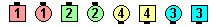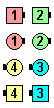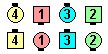\$B\$NA0(B As Couples 1/2 Tag\$B\$N8e(B 1/2 Tag\$B\$N8e(B (\$B=*\$o\$j(B)
Echo Tandem Hinge: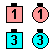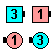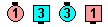\$B\$NA0(B Tandem Hinge\$B\$N8e(B Hinge\$B\$N8e(B (\$B=*\$o\$j(B)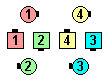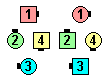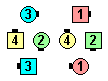\$B\$NA0(B 6 By 2 Acey Deucey\$B\$N8e(B Acey Deucey\$B\$N8e(B (\$B=*\$o\$j(B)
Echo Tandem Twosome Cast Off 3/4: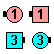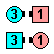\$B\$NA0(B Tandem Twosome Cast Off 3/4\$B\$N8e(B Cast Off 3/4\$B\$N8e(B (\$B=*\$o\$j(B)

\$BCm
• Echo \$B\$N8@MU\$r\$H\$j=|\$\$\$F%3!<%kA4BN\$r9T\$\$(B; Echo \$B\$N8@MU\$H\$=\$NONCEPT \$B\$r\$H\$j=|\$\$\$F%3!<%k\$r\$b\$&0lEY9T\$\$\$^\$9(B.
• \$BNc\$(\$P(B, Echo Cross Concentric Spin The Top \$B\$O(B Cross Concentric Spin The Top \$B\$r\$7\$F(B, Spin The Top \$B\$r\$7\$^\$9(B (Cross Concentric \$B\$N%3%s%;%W%H\$,\$J\$/\$J\$j\$^\$9(B, Cross \$B\$N8@MU\$r\$J\$/\$9\$N\$G\$O\$"\$j\$^\$;\$s(B).
• \$B;O\$a\$N%3%s%;%W%H\$@\$1\$r\$H\$j=|\$/\$H\$\$\$&\$3\$H\$KCm0U\$7\$F\$/\$@\$5\$\$(B. \$BNc\$(\$P(B, \$B:8\$N(B Column \$B\$+\$i(B Split Phantom Column Circulate Twice \$B\$r\$7\$^\$9(B. \$B\$=\$7\$F\$=\$3\$+\$i(B, Echo Split Phantom Waves Tandem Spin The Top \$B\$r9M\$(\$F\$_\$^\$7\$g\$&(B. \$B\$3\$N>l9g(B, Split Phantom Waves Tandem Spin The Top \$B\$r\$7\$F(B, Tandem Spin The Top \$B\$r\$7\$^\$9(B.
• \$B%Q!<%H\$K\$+\$+\$o\$k%3%s%;%W%H\$H0l=o\$K;H\$&\$H(B, Echo \$B\$O5^\$KFq\$7\$/\$J\$j\$^\$9(B.

\$B (\$B1&\$N(B Column \$B\$+\$i(B):

• Echo Tandem 1/4 Thru:
1.  Tandem 1/4 Thru;
2.  1/4 Thru.
• Echo Initially Tandem 1/4 Thru:
1.  Initially Tandem 1/4 Thru;
2.  1/4 Thru.
\$B\$3\$N(B 2 \$B\$DL\\$NNc\$K\$O[#Kf\$5\$,\$"\$j(B, \$B%3!<%i!<\$N%3!<%k\$N;EJ}\$K\$h\$C\$F\$O(B, Initially \$B\$@\$1\$r\$H\$j=|\$3\$&\$H\$9\$k\$3\$H\$,\$"\$jF@\$^\$9(B. Initially \$B\$NJ}\$r\$H\$j=|\$/\$Y\$-\$H9M\$(\$^\$9(B. \$B\$3\$l\$O(B, \$BA0\$K=R\$Y\$?(B Echo Cross Concentric \$B\$HF1\$8\$3\$H\$G\$9(B.
• Initially Echo Tandem 1/4 Thru:
1.  Echo Tandem Right Arm Turn 1/4 (Tandem Right Arm Turn 1/4 \$B\$r\$7\$F(B Right Arm Turn 1/4)
2.  (Centers) Left Arm Turn 1/2.
• \$B%3!<%i!<\$X\$NDs0F(B: C3B \$B\$G\$O(B, Echo \$B\$r%Q!<%HJ,\$1\$K\$+\$+\$o\$k%3%s%;%W%H\$H0l=o\$K\$O;H\$o\$J\$\$\$h\$&\$K\$7\$F\$/\$@\$5\$\$(B. C4 \$B\$G\$N\$_(B, \$B\$R\$+\$(\$a\$K;H\$C\$F\$/\$@\$5\$\$(B.

Echo \$B\$N0J2<\$N3HD%\$,(B C4 \$B\$G;H\$o\$l\$F\$\$\$^\$9(B:

• Double Echo Concept1 Concept2 {anything} [C4]:

• \$B0J2<\$r9T\$\$\$^\$9(B:
1.  Concept1 Concept2 {anything};
2.  Concept2 {anything};
3.  {anything}.
• \$BNc(B, \$B1&\$N(B Column \$B\$+\$iA40w(B Squeeze \$B\$r\$7\$?\$H\$3\$m\$+\$i(B: Double Echo Split Phantom Columns Tandem Scoot Back: Split Phantom Columns Tandem Scoot Back; Tandem Scoot Back; Scoot Back.
• Triple Echo Concept1 Concept2 Concept3 {anything} [C4]:

• \$B0J2<\$r9T\$\$\$^\$9(B:
1.  Concept1 Concept2 Concept3 {anything};
2.  Concept2 Concept3 {anything};
3.  Concept3 {anything};
4.  {anything}.
• Quadruple Echo Concept1 Concept2 Concept3 Concept4 {anything} [C4]:

• \$B0J2<\$r9T\$\$\$^\$9(B:
1.  Concept1 Concept2 Concept3 Concept4 {anything};
2.  Concept2 Concept3 Concept4 {anything};
3.  Concept3 Concept4 {anything};
4.  Concept4 {anything};
5.  {anything}.
• Reverse Echo {any CONCEPT} {anything} [C4]:

• \$B0J2<\$r9T\$\$\$^\$9(B:
1.  {anything};
2.  Concept {anything}.
• \$BNc(B, Tidal Wave \$B\$+\$i(B, Reverse Echo Tandem Spin The Top: Spin The Top; Tandem Spin The Top.